# 7.10: Exercise Supplement

$$\newcommand{\vecs}{\overset { \rightharpoonup} {\mathbf{#1}} }$$ $$\newcommand{\vecd}{\overset{-\!-\!\rightharpoonup}{\vphantom{a}\smash {#1}}}$$$$\newcommand{\id}{\mathrm{id}}$$ $$\newcommand{\Span}{\mathrm{span}}$$ $$\newcommand{\kernel}{\mathrm{null}\,}$$ $$\newcommand{\range}{\mathrm{range}\,}$$ $$\newcommand{\RealPart}{\mathrm{Re}}$$ $$\newcommand{\ImaginaryPart}{\mathrm{Im}}$$ $$\newcommand{\Argument}{\mathrm{Arg}}$$ $$\newcommand{\norm}{\| #1 \|}$$ $$\newcommand{\inner}{\langle #1, #2 \rangle}$$ $$\newcommand{\Span}{\mathrm{span}}$$ $$\newcommand{\id}{\mathrm{id}}$$ $$\newcommand{\Span}{\mathrm{span}}$$ $$\newcommand{\kernel}{\mathrm{null}\,}$$ $$\newcommand{\range}{\mathrm{range}\,}$$ $$\newcommand{\RealPart}{\mathrm{Re}}$$ $$\newcommand{\ImaginaryPart}{\mathrm{Im}}$$ $$\newcommand{\Argument}{\mathrm{Arg}}$$ $$\newcommand{\norm}{\| #1 \|}$$ $$\newcommand{\inner}{\langle #1, #2 \rangle}$$ $$\newcommand{\Span}{\mathrm{span}}$$$$\newcommand{\AA}{\unicode[.8,0]{x212B}}$$

## Exercise Supplement

### Graphing Linear Equations and Inequalities in One Variable

For the following problems, graph the equations and inequalities.

##### Exercise $$\PageIndex{1}$$

$$6x−18=6$$$$x=4$$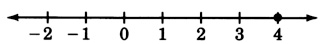##### Exercise $$\PageIndex{2}$$

$$4x−3=−7$$##### Exercise $$\PageIndex{3}$$

$$5x−1=2$$$$x = \dfrac{3}{5}$$##### Exercise $$\PageIndex{4}$$

$$10x−16<4$$##### Exercise $$\PageIndex{5}$$

$$−2y+1≤5$$$$y≥−2$$

##### Exercise $$\PageIndex{6}$$

$$\dfrac{-7a}{12} \ge 2$$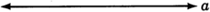##### Exercise $$\PageIndex{7}$$

$$3x+4≤12$$$$x \le \dfrac{8}{3}$$##### Exercise $$\PageIndex{8}$$

$$−16≤5x−1≤−11$$##### Exercise $$\PageIndex{9}$$

$$0<−3y+9≤9$$$$0≤y<3$$##### Exercise $$\PageIndex{10}$$

$$\dfrac{-5c}{2} + 1 = 7$$### Plotting Points in the Plane

##### Exercise $$\PageIndex{11}$$

Draw a coordinate system and plot the following ordered pairs.

$$(3, 1), (4, -2), (-1, -3), (0, 3), (3, 0), (5, -\dfrac{2}{3})$$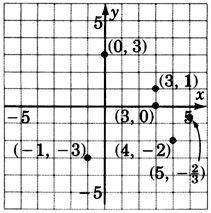##### Exercise $$\PageIndex{12}$$

As accurately as possible, state the coordinates of the points that have been plotted on the graph.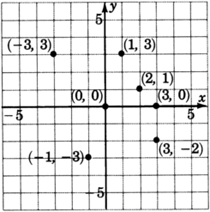### Graphing Linear Equations in Two Variables

##### Exercise $$\PageIndex{13}$$

What is the geometric structure of the graph of all the solutions to the linear equation $$y=4x−9$$?

a straight line

### Graphing Linear Equations in Two Variables - Graphing Equations in Slope-Intercept Form

For the following problems, graph the equations.

##### Exercise $$\PageIndex{14}$$

$$y−x=2$$

##### Exercise $$\PageIndex{15}$$

$$y+x−3=0$$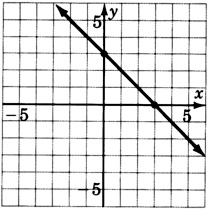##### Exercise $$\PageIndex{16}$$

$$−2x+3y=−6$$

##### Exercise $$\PageIndex{17}$$

$$2y+x−8=0$$##### Exercise $$\PageIndex{18}$$

$$4(x−y)=12$$

##### Exercise $$\PageIndex{19}$$

$$3y−4x+12=0$$##### Exercise $$\PageIndex{20}$$

$$y=−3$$

##### Exercise $$\PageIndex{21}$$

$$y−2=0$$##### Exercise $$\PageIndex{22}$$

$$x=4$$

##### Exercise $$\PageIndex{23}$$

$$x+1=0$$##### Exercise $$\PageIndex{24}$$

$$x=0$$

##### Exercise $$\PageIndex{25}$$

$$y=0$$### The Slope-Intercept Form of a Line

##### Exercise $$\PageIndex{26}$$

Write the slope-intercept form of a straight line.

##### Exercise $$\PageIndex{27}$$

The slope of a straight line is a ____ of the steepness of the line.

measure

##### Exercise $$\PageIndex{28}$$

Write the formula for the slope of a line that passes through the points $$(x_1,y_1)$$ and $$(x_2,y_2)$$.

For the following problems, determine the slope and y-intercept of the lines.

##### Exercise $$\PageIndex{29}$$

$$y=4x+10$$

slope:  $$4$$

$$y$$-intercept:  $$(0,10)$$

##### Exercise $$\PageIndex{30}$$

$$y=3x−11$$

##### Exercise $$\PageIndex{31}$$

$$y=9x−1$$

slope:  $$9$$

$$y$$-intercept:  $$(0,-1)$$

##### Exercise $$\PageIndex{32}$$

$$y=−x+2$$

##### Exercise $$\PageIndex{33}$$

$$y=−5x−4$$

slope:  $$-5$$

$$y$$-intercept:  $$(0,-4)$$

##### Exercise $$\PageIndex{34}$$

$$y=x$$

##### Exercise $$\PageIndex{35}$$

$$y=−6x$$

slope:  $$-6$$

$$y$$-intercept:  $$(0,0)$$

##### Exercise $$\PageIndex{36}$$

$$3y=4x+9$$

##### Exercise $$\PageIndex{37}$$

$$4y=5x+1$$

slope:  $$\dfrac{5}{4}$$

$$y$$-intercept:  $$(0,\dfrac{1}{4})$$

##### Exercise $$\PageIndex{38}$$

$$2y=9x$$

##### Exercise $$\PageIndex{39}$$

$$5y+4x=6$$

slope:  $$-\dfrac{4}{5}$$

$$y$$-intercept:  $$(0,\dfrac{6}{5})$$

##### Exercise $$\PageIndex{40}$$

$$7y+3x=10$$

##### Exercise $$\PageIndex{41}$$

$$6y−12x=24$$

slope:  $$2$$

$$y$$-intercept:  $$(0,4)$$

##### Exercise $$\PageIndex{42}$$

$$5y−10x−15=0$$

##### Exercise $$\PageIndex{43}$$

$$3y+3x=1$$

slope:  $$-1$$

$$y$$-intercept:  $$(0,\dfrac{1}{3})$$

##### Exercise $$\PageIndex{44}$$

$$7y+2x=0$$

##### Exercise $$\PageIndex{45}$$

$$y=4$$

slope:  $$0$$

$$y$$-intercept:  $$(0,4)$$

For the following problems, find the slope, if it exists, of the line through the given pairs of points.

##### Exercise $$\PageIndex{46}$$

$$(5,2),(6,3)$$

##### Exercise $$\PageIndex{47}$$

$$(8,−2),(10,−6)$$

slope: $$−2$$

##### Exercise $$\PageIndex{48}$$

$$(0,5),(3,4)$$

##### Exercise $$\PageIndex{49}$$

$$(1,−4),(3,3)$$

slope: $$\dfrac{7}{2}$$

##### Exercise $$\PageIndex{50}$$

$$(0,0),(−8,−5)$$

##### Exercise $$\PageIndex{51}$$

$$(−6,1),(−2,7)$$

slope:  $$\dfrac{3}{2}$$

##### Exercise $$\PageIndex{52}$$

$$(−3,−2),(−4,−5)$$

##### Exercise $$\PageIndex{53}$$

$$(4,7),(4,−2)$$

No Slope

##### Exercise $$\PageIndex{54}$$

$$(−3,1),(4,1)$$

##### Exercise $$\PageIndex{55}$$

$$(\dfrac{1}{3}, \dfrac{3}{4}), (\dfrac{2}{9}, -\dfrac{5}{6})$$

slope: $$\dfrac{57}{4}$$

##### Exercise $$\PageIndex{56}$$

Moving left to right, lines with slope rise while lines with slope decline.

##### Exercise $$\PageIndex{57}$$

Compare the slopes of parallel lines.

The slopes of parallel lines are equal.

### Finding the Equation of a Line

For the following problems, write the equation of the line using the given information. Write the equation in slope-intercept form.

##### Exercise $$\PageIndex{58}$$

Slope=$$4$$, $$y$$-intercept=$$5$$

##### Exercise $$\PageIndex{59}$$

Slope=$$3$$, $$y$$-intercept=$$-6$$

$$y=3x−6$$

##### Exercise $$\PageIndex{60}$$

Slope=$$1$$, $$y$$-intercept=$$8$$

##### Exercise $$\PageIndex{61}$$

Slope=$$1$$, $$y$$-intercept=$$-2$$

$$y=x−2$$

##### Exercise $$\PageIndex{62}$$

Slope=$$-5$$, $$y$$-intercept=$$1$$

##### Exercise $$\PageIndex{63}$$

Slope=$$-11$$, $$y$$-intercept=$$-4$$

$$y=−11x−4$$

##### Exercise $$\PageIndex{64}$$

Slope=$$2$$, $$y$$-intercept=$$0$$

##### Exercise $$\PageIndex{65}$$

Slope=$$-1$$, $$y$$-intercept=$$0$$

$$y=−x$$

##### Exercise $$\PageIndex{66}$$

$$m=3,(4,1)$$

##### Exercise $$\PageIndex{67}$$

$$m=2,(1,5)$$

$$y=2x+3$$

##### Exercise $$\PageIndex{68}$$

$$m=6,(5,−2)$$

##### Exercise $$\PageIndex{69}$$

$$m=−5,(2,−3)$$

$$y=−5x+7$$

##### Exercise $$\PageIndex{70}$$

$$m=−9,(−4,−7)$$

##### Exercise $$\PageIndex{71}$$

$$m=−2,(0,2)$$

$$y=−2x+2$$

##### Exercise $$\PageIndex{72}$$

$$m=−1,(2,0)$$

##### Exercise $$\PageIndex{73}$$

$$(2,3),(3,5)$$

$$y=2x−1$$

##### Exercise $$\PageIndex{74}$$

$$(4,4),(5,1)$$

##### Exercise $$\PageIndex{75}$$

$$(6,1),(5,3)$$

$$y=−2x+13$$

##### Exercise $$\PageIndex{76}$$

$$(8,6),(7,2)$$

##### Exercise $$\PageIndex{77}$$

$$(−3,1),(2,3)$$

$$y = \dfrac{2}{5}x + \dfrac{11}{5}$$

##### Exercise $$\PageIndex{78}$$

$$(−1,4),(−2,−4)$$

##### Exercise $$\PageIndex{79}$$

$$(0,−5),(6,−1)$$

$$y = \dfrac{2}{3}x - 5$$

##### Exercise $$\PageIndex{80}$$

$$(2,1),(6,1)$$

##### Exercise $$\PageIndex{81}$$

$$(−5,7),(−2,7)$$

$$y=7$$ (zero slope)

##### Exercise $$\PageIndex{82}$$

$$(4,1),(4,3)$$

##### Exercise $$\PageIndex{83}$$

$$(−1,−1),(−1,5)$$

$$x=−1$$ (no slope)

##### Exercise $$\PageIndex{84}$$

$$(0,4),(0,−3)$$

##### Exercise $$\PageIndex{85}$$

$$(0,2),(1,0)$$

$$y=−2x+2$$

For the following problems, reading only from the graph, determine the equation of the line.

##### Exercise $$\PageIndex{86}$$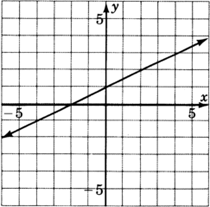##### Exercise $$\PageIndex{87}$$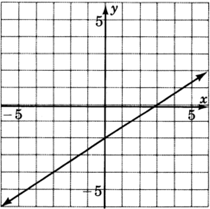$$y = \dfrac{2}{3}x - 2$$

##### Exercise $$\PageIndex{88}$$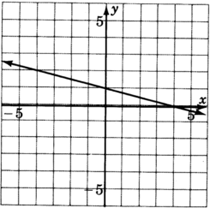##### Exercise $$\PageIndex{89}$$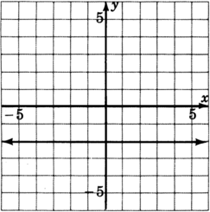$$y=−2$$

##### Exercise $$\PageIndex{90}$$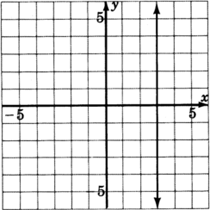##### Exercise $$\PageIndex{91}$$$$y=1$$

### Graphing Linear Inequalities in Two Variables

For the following problems, graph the inequalities.

##### Exercise $$\PageIndex{92}$$

$$y≤x+2$$##### Exercise $$\PageIndex{93}$$

$$y < -\dfrac{1}{2} + 3$$##### Exercise $$\PageIndex{94}$$

$$y > \dfrac{1}{3}x - 3$$##### Exercise $$\PageIndex{95}$$

$$−2x+3y≤−6$$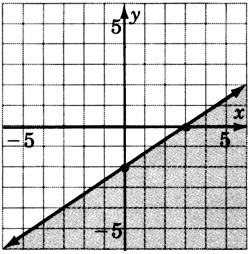##### Exercise $$\PageIndex{96}$$

$$2x+5y≥20$$##### Exercise $$\PageIndex{97}$$

$$4x−y+12>0$$##### Exercise $$\PageIndex{98}$$

$$y≥−2$$##### Exercise $$\PageIndex{99}$$

$$x<3$$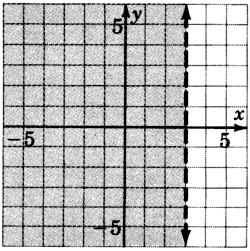##### Exercise $$\PageIndex{100}$$
$$y≤0$$